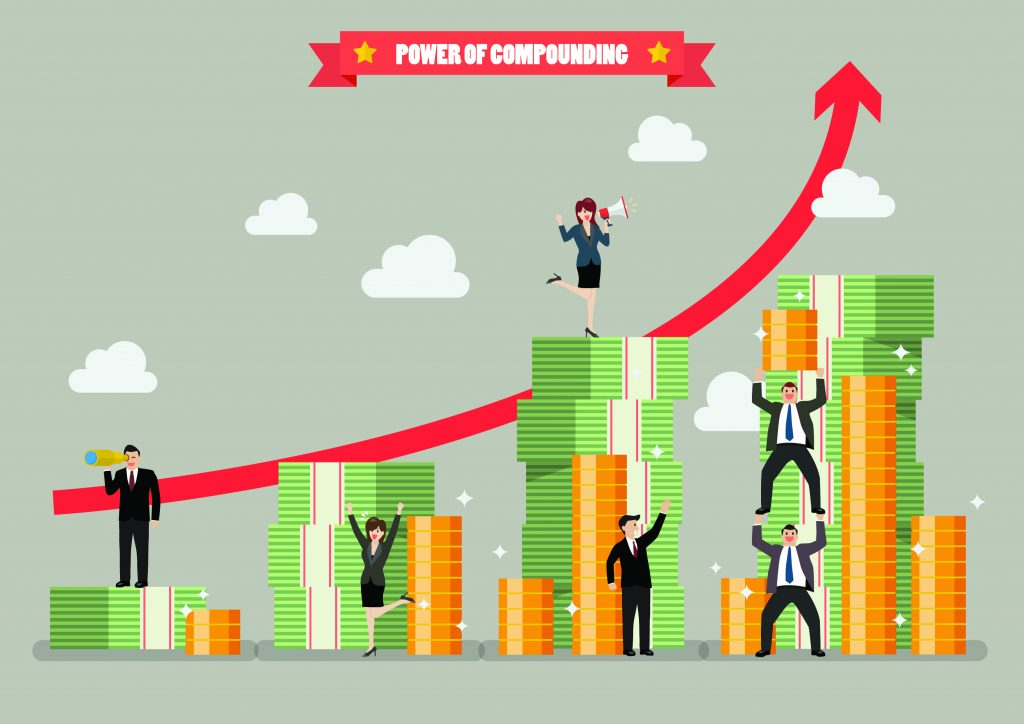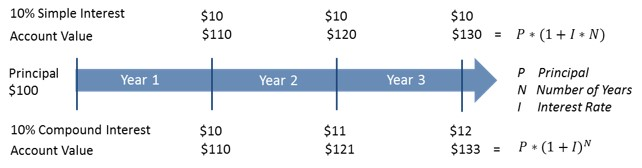# Power of Compounding

## An investment of \$100 growing at a 10% annual compounded rate will be \$1.4M in 100 years!

This basic statement expresses the earning power of compounded interest on an initial investment amount.  How so?

First, let’s understand how simple interest works. Simple interest is only paid on the principal or original investment amount. Hence, the same amount of interest is paid over each interest period no matter how long the investment is kept.

With compound interest, interest is paid on both the principal and past interest earnings, with the result that an increasing amount of interest is earned with each successive interest period. The compounding effect in the increasing amount of earned interest results in a substantially larger account value.In the given example, simple interest generated is always \$10 each year.  Compound interest instead grows from \$10 to \$12 in two annual interest cycles. The compound interest formula includes time period in the exponent factor (rather than as a multiple factor like in simple interest), which produces the accelerated growth.

We can now further demonstrate the power of compound interest over a long investment period. If we let \$100 grow at 10% CAGR (Compound Annual Growth Rate) for 100 years, the end balance will be \$1.4M. Compare this to the simple interest example in which the end balance is a mere \$1,100.

The exponential growth effect of compounding interest does not manifest until the latter years of investment growth. Any premature withdrawal from the investment will not produce a return that is anything close to the return on an investment held to its full term. In terms of our example: compare \$10K at the end of 50 years to \$1.4M at the end of 100 years.

Notice how a point change in interest rate makes a great difference in the respective end balances. The longer the term of investment, the greater the difference between dollar values. However, expressed in multiples (or percentage terms), the end balance changes by ≈2.5x (or ≈150%) for every point change in CAGR for a 100-year investment period. Let us work out the multiples for more practical time periods.

The table numerically shows the diverging effect that occurs between the CAGR curves in the viewgraph. The effect of CAGR change is more pronounced and accelerated as the investment time horizon expands. In summary, a point change in CAGR causes the end balance to change 10% points per decade of investment. Find a more practical application of compounding in “Asset Growth Using Rule of 72”.

We specialize in tax-free retirement strategy and investments such as IUL, Annuity and LTC. Prefer a quick and complimentary consultation? Just email us at Consult@FinCrafters.com!